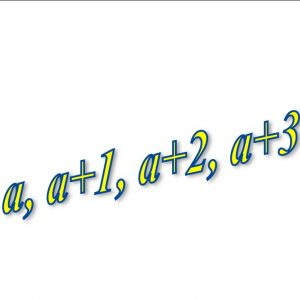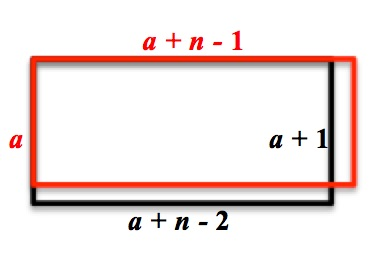Choose four consecutive whole numbers, for example, 4, 5, 6 and 7.
Multiply the first and last numbers together (28).
Multiply the middle pair together (30).
Choose different sets of four consecutive whole numbers and do the same.
What do you notice about the difference between the two products each time?

Choose five consecutive whole numbers, for example, 3, 4, 5, 6 and 7.
Multiply the first and last numbers together (21).
Multiply the second and fourth numbers together (24).
Choose different sets of five consecutive whole numbers and do the same.
What do you notice now about the difference between the two products?What happens when you take 6 or 7 or 8 , or … n consecutive whole numbers
and compare the product of the first and last numbers
with the product of the second and penultimate numbers?